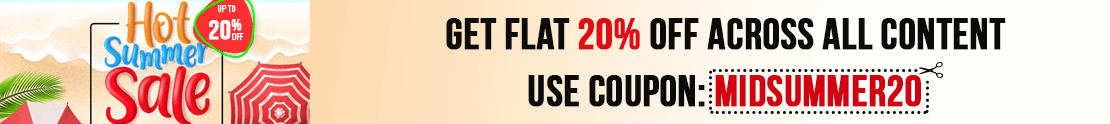# User ForumSubject :IMO    Class : Class 3

## Ans 1:

Class : Class 3

Subject :IMO    Class : Class 2

## Ans 1:

Class : Class 2
Length of pen P = 10 Length of pen Q = 7 Length of pen R = 4 Length of pen S = 9 So, the required arrangement of pens fromthe longest to the shortest is P, S, Q, R.

Subject :IMO    Class : Class 2

## Ans 1:

Class : Class 2
P. 182 246 134 = 562â´ Digit at tens place is 6.Q. 425 367 156 = 948â´ Digit at tens place is 4.R. 142 464 292 = 898â´ Digit at tens place is 9.S. 325 134 272 = 731â´ Digit at tens place is 3.â´ P â c, Q â a, R â d, S â b

## Ans 2:

Class : Class 2
P. 182 246 134 = 562â´ Digit at tens place is 6.Q. 425 367 156 = 948â´ Digit at tens place is 4.R. 142 464 292 = 898â´ Digit at tens place is 9.S. 325 134 272 = 731â´ Digit at tens place is 3.â´ P â c, Q â a, R â d, S â b

Subject :IMO    Class : Class 5

## Ans 1:

Class : Class 6
A

Subject :IMO    Class : Class 8

Subject :IMO    Class : Class 3

## Ans 1:

Class : Class 3
S,R,Q,P

Subject :IMO    Class : Class 1

## Ans 1:

Class : Class 2
C

Subject :IMO    Class : Class 1

## Ans 1:

Class : Class 2
C

Subject :IMO    Class : Class 1